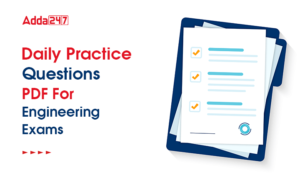Engineering Jobs   »   QUIZ: CIVIL ENGINEERING (21-APRIL-2020)

# QUIZ: CIVIL ENGINEERING (21-APRIL-2020)

Quiz: Civil Engineering
Exam: UPSSSC-JE
Topic: Reinforced Concrete Cement (RCC)

Each question carries 1 mark
Negative marking: 1/4 mark
Time: 10 Minutes

Q1. Shear reinforcement in a RCC beam is provided to resist
(a) Diagonal tension
(b) Bending stress
(c) Compressive stress
(d) Punching shear

Q2. Diagonal tension reinforcement is provided in a beam as
(a) longitudinal bars
(b) bent up bars and vertical stirrups
(c) helical reinforcement
(d) 90° bend at the bend of main bar

Q3. The bond strength of concrete increases with:
(a) The quantity of steel
(b) The tensile strength of steel
(d) the quantity of concrete

Q4. Lap length in compression shall not be less than
(a) 15 ϕ
(b) 20 ϕ
(c) 24 ϕ
(d) 30 ϕ

Q5. If ‘d’ is the diameter of the steel bar, diameter of hook is _______
(a) 2d
(b) 4d
(c) 6d
(d) 8d

Q6. In reinforced concrete, lap splice should not be used for bars having diameter larger than
(a) 36 mm
(b) 25 mm
(c) 32 mm
(d) 30 mm

Q7. If nominal shear stress (τ_v) exceeds the design shear strength of concrete (τ_C), the shear reinforcement as per IS – 456 will be provided for shear equal to –
(a) τ_C
(b) τ_v
(c) τ_v- τ_C
(d) τ_v+ τ_C

Q8. The nominal shear stress (τ_v) in a reinforced concrete beam is given by
(a) bd/V_u
(b) V_u/(bd )
(c) V_u bd
(d) (V_u.b)/d

Q9. Diagonal tension in beam…………..
(a) Is maximum at neutral axis
(b) Decreases below the neutral axis and increases above the neutral axis
(c) Increases below the neutral axis and decreases above the neutral axis
(d) Remains the same in both above and below the neutral axis

Q10. In limit state method of design, for bars in compression the values of bond stress shall be:
(a) Decreased by 20%
(b) Increased by 25%
(c) Decreased by 25%
(d) Increased by 20%

Solutions

S1. Ans.(a)
Sol. shear reinforcement in RCC beam is provided to resist diagonal tension. Stirrups is provided in the beam for shear reinforcement. At corner spacing between stirrups decreases and increase towards centre.

S2. Ans.(b)
Sol. Diagonal tension reinforcement is provided in the form of-
Vertical stirrups
Inclined stirrups
Bent up bars.

S3. Ans.(c)
Sol. bond strength of concrete depends on-
Frictional resistance
Mechanical resistance
→ if grade of concrete increases then friction b/w concrete & steel increases.
S4. Ans.(c)
Sol. → (Lap length in flexure tension)/(Ld (or)30ϕ)_greater
→ (Lap length in Direct tension)/(2Ld (or)30ϕ)_greater
→ (Lap length in compression)/(Ld (or)24ϕ)_greater
S5. Ans.(b)
Sol. if ‘d’ is diameter of steel bar then diameter of hook is ‘4d’

S6. Ans.(a)
Sol. if diameter of bar greater than 36 mm than welding should be done instead of lap splices.

S7. Ans.(c)
Sol. if nominal shear stress (τ_v ) exceceds the design shear strength of concrete (τ_c ) than shear reinforcement will be provided to resist (τ_v-τ_c )
Some important point in shear reinforcement
→ if τ_V> τ_cmax then redesign the section
→if τ_V<τ_c/2 then no shear reinforcement provided
→ if τ_V < τ_c minimum shear reinforcement provided
→ if τ_V > τ_c then design shear reinforcement to resist (τ_V-τ_c )
S8. Ans.(b)
Sol. Nominal Shear stress (τ_v )=V_u/(bd )
V_u→shear force
bd→cross sectional area.

S9. Ans.(c)
Sol. Diagonal tension in beam increase below neutral axis & decrease above neutral axis.

S10. Ans.(b)
Sol. Table of bond stress in IS code given for plain bars in tension.
→ for HYSD bar in tension increase the value by 60%
→ for bar’s in compression the value is increased by 25%

Sharing is caring!

•GATE Result 2023 Out, Download Result, C...
•GATE 2023 Admit Card Out, Download Link ...
•UKPSC Draftsman Syllabus 2023 and Exam P...
•UP Polytechnic Exam Date 2023 Out, Downl...
•JSSC JE Syllabus 2023, Check Latest Exam...
•Daily Practice Questions PDF For Enginee...
•ISRO Recruitment 2023, Latest Notificati...
•UPSC ESE Previous Year Papers, Download ...